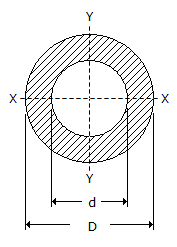Courses

# Test: Engineering Mechanics - 2

## 25 Questions MCQ Test Engineering Mechanics | Test: Engineering Mechanics - 2

Description
This mock test of Test: Engineering Mechanics - 2 for Mechanical Engineering helps you for every Mechanical Engineering entrance exam. This contains 25 Multiple Choice Questions for Mechanical Engineering Test: Engineering Mechanics - 2 (mcq) to study with solutions a complete question bank. The solved questions answers in this Test: Engineering Mechanics - 2 quiz give you a good mix of easy questions and tough questions. Mechanical Engineering students definitely take this Test: Engineering Mechanics - 2 exercise for a better result in the exam. You can find other Test: Engineering Mechanics - 2 extra questions, long questions & short questions for Mechanical Engineering on EduRev as well by searching above.
QUESTION: 1

Solution:
QUESTION: 2

Solution:
QUESTION: 3

### A body will begin to move down an inclined plane if the angle of inclination of the plane is __________ the angle of friction.

Solution:
QUESTION: 4

When a particle moves along a circular path with uniform velocity, there will be no tangential acceleration.

Solution:
QUESTION: 5

The bodies which rebound after impact are called

Solution:
QUESTION: 6

The maximum frictional force, which comes into play, when a body just begins to slide over the surface of the other body, is known as

Solution:
QUESTION: 7

The centre of gravity of an equilateral triangle with each side a, is __________ from any of the three sides.

Solution:
QUESTION: 8

The distance, between the point of projection and the point where the projectile strikes the ground, is known as range.

Solution:
QUESTION: 9

The triangle law of forces states that if two forces acting simultaneously on a particle, be represented in magnitude and direction by the two sides of a triangle taken in order, then their resultant may be represented in magnitude and direction by the third side of a triangle, taken in opposite order.

Solution:
QUESTION: 10

The algebraic sum of the resolved parts of a number of forces in a given direction is equal to the resolved part of their resultant in the same direction. This is known as

Solution:
QUESTION: 11

The path of the projectile is a parabola.

Solution:
QUESTION: 12

The angle between two forces when the resultant is maximum and minimum respectively are

Solution:
QUESTION: 13

The equivalent length of a simple pendulum which gives the same frequency as compound pendulum is

Solution:
QUESTION: 14

A redundant frame is also called __________ frame.

Solution:
QUESTION: 15

Mass moment of inertia of a uni form thin rod of mass M and length (l) about its mid-point and perpendicular to its length is

Solution:
QUESTION: 16

A resultant force is a single force which produces the same effect as produced by all the given forces acting on a body.

Solution:
QUESTION: 17

When a rigid body is suspended vertically, and it oscillates with a small amplitude under the action of the force of gravity, the body is known as

Solution:
QUESTION: 18

Moment of inertia of a hollow circular section, as shown in the below figure about X-axis, isSolution:
QUESTION: 19

If two blocks of equal mass are attached to the two ends of a light string and one of the blocks is placed over a smooth horizontal plane while the other is hung freely after passing over a smooth pulley, then the two blocks will have some motion.

Solution:
QUESTION: 20

During elastic impact, the relative velocity of the two bodies after impact is __________ the relative velocity of the two bodies before impact.

Solution:
QUESTION: 21

Work done is said to be zero, when

Solution:
QUESTION: 22

The force which acts along the radius of a circle and directed away from the centre of the circle is called centrifugal force.

Solution:
QUESTION: 23

One watt is equal to

Solution:
QUESTION: 24

two like parallel forces are acting at a distance of 24 mm apart and their resultant is 20 N. It the line of action of the resultant is 6 mm from any given force, the two forces are

Solution:
QUESTION: 25

If two bodies having masses m1 and m2 (m1>m2) have equal kinetic energies, the momentum of body having mass m1 is __________ the momentum of body having mass m2.

Solution: